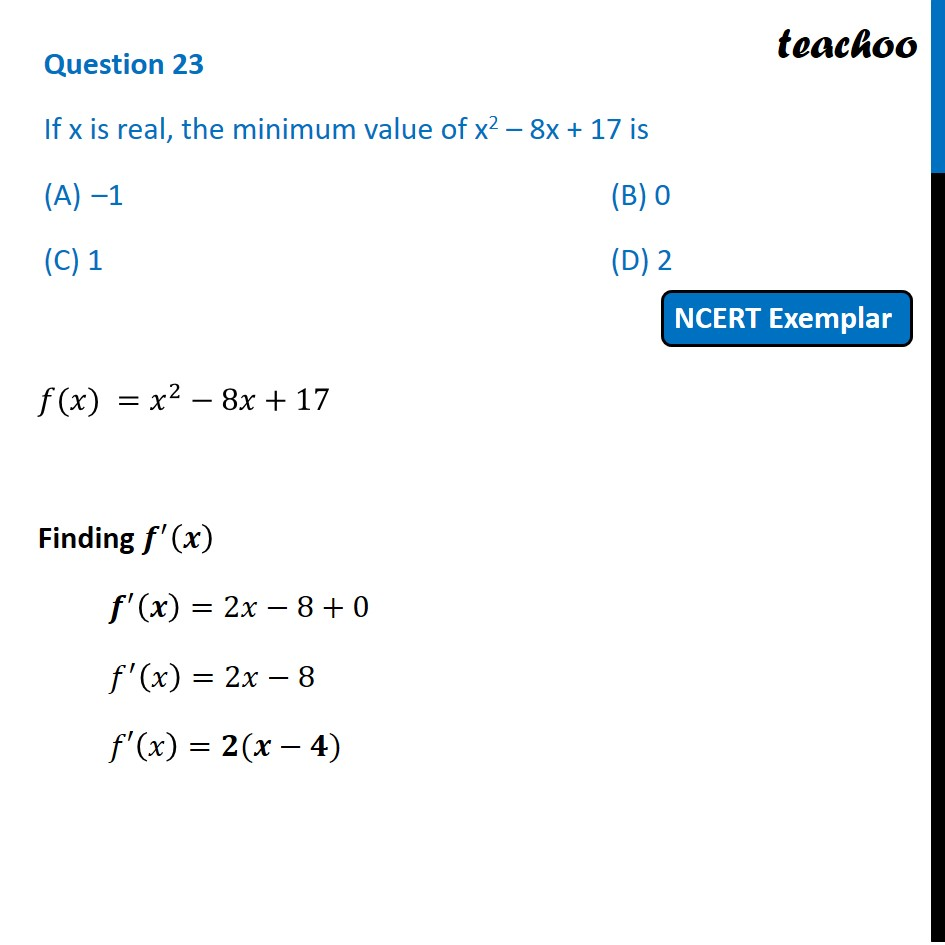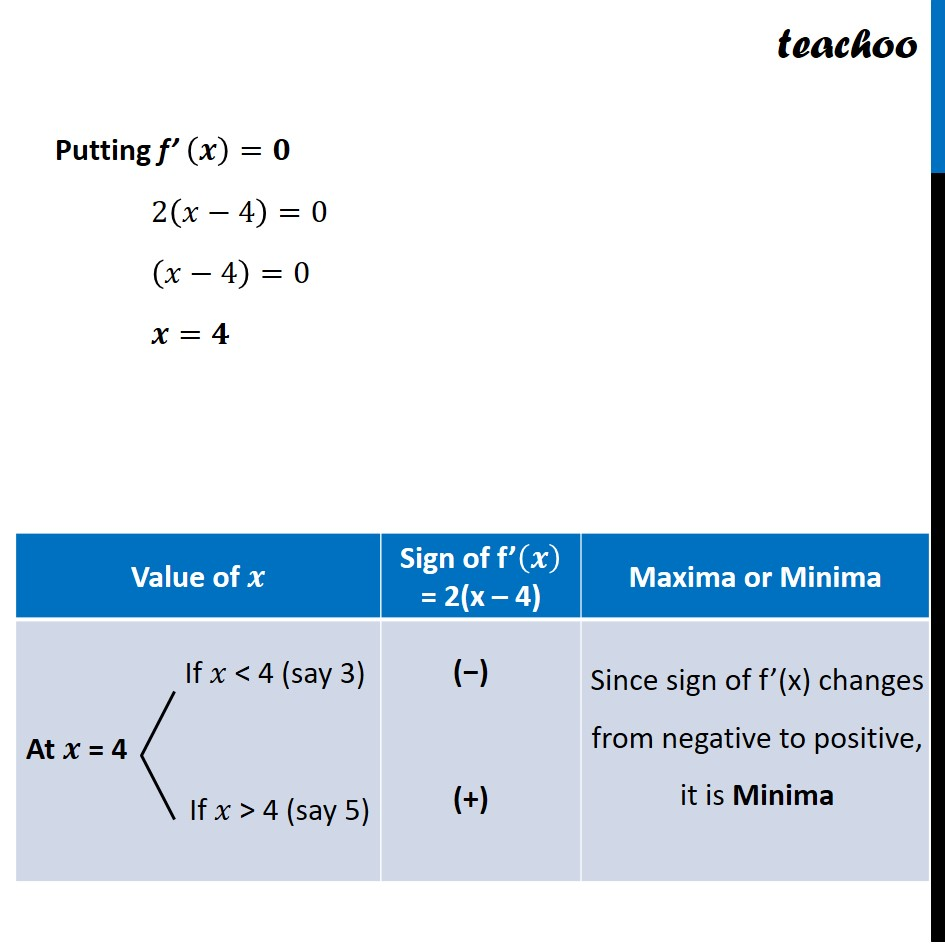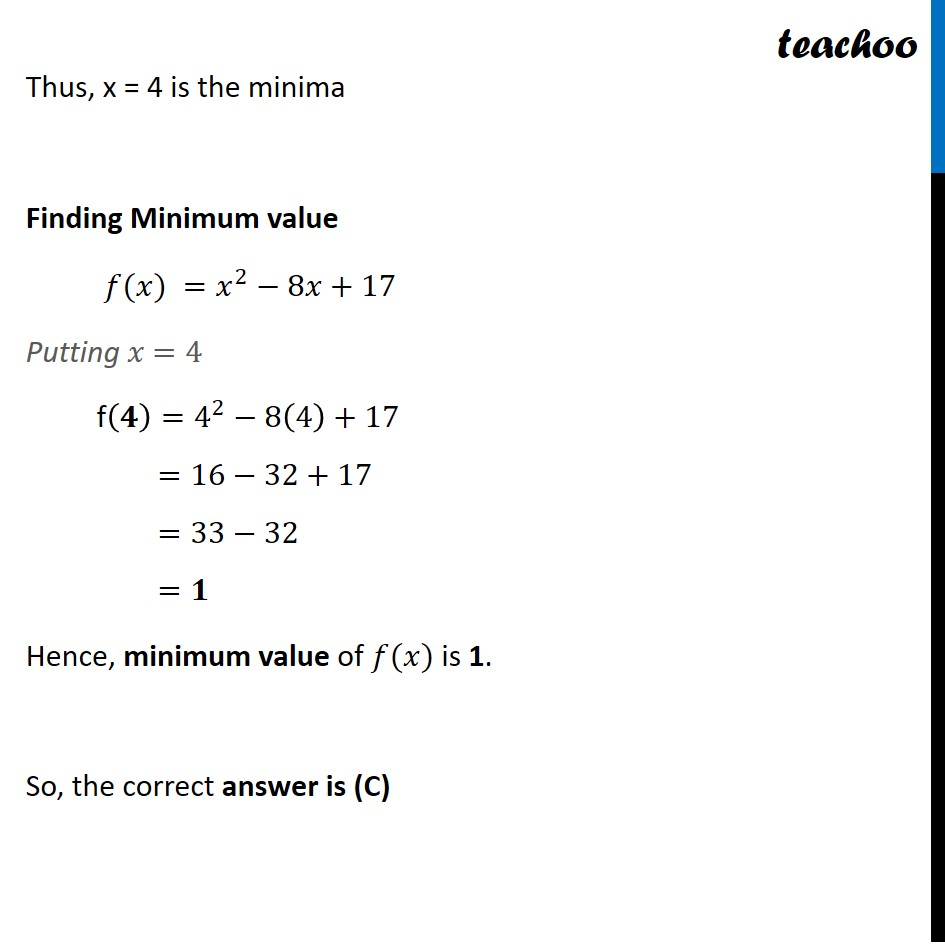NCERT Exemplar - MCQs

Chapter 6 Class 12 Application of Derivatives
Serial order wise

## (C) 1                                 (D) 2

This question is similar to Ex 6.5, 1 (i) - Chapter 6 Class 12 - Application of DerivativesLearn in your speed, with individual attention - Teachoo Maths 1-on-1 Class

### Transcript

Question 10 If x is real, the minimum value of x2 – 8x + 17 is –1 (B) 0 (C) 1 (D) 2 𝑓(𝑥) =𝑥^2−8𝑥+17 Finding 𝒇^′ (𝒙) 𝒇^′ (𝒙)=2𝑥−8+0 𝑓^′ (𝑥)=2𝑥−8 𝑓′(𝑥)=𝟐(𝒙−𝟒) Putting f’ (𝒙)=𝟎 2(𝑥−4)=0 (𝑥−4)=0 𝒙=𝟒 Thus, x = 4 is the minima Finding Minimum value 𝑓(𝑥) =𝑥^2−8𝑥+17 Putting 𝑥 =4 f(𝟒)=4^2−8(4)+17 =16−32+17 =33−32 =𝟏 Hence, minimum value of 𝑓(𝑥) is 1. So, the correct answer is (C)